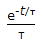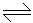# Chemical Engineering - Chemical Reaction Engineering

### Exercise :: Chemical Reaction Engineering - Section 8

1.

For the same residence time, which one will give the maximum conversion ?

 A. Single stirred tank (v = 5 litres). B. Two stirred tank (each of 2.5 litres) in series. C. Stirred tank followed by tubular flow reactor (each of 2.5 litres). D. Single tubular flow reactor (v = 5 litres).

Explanation:

No answer description available for this question. Let us discuss.

2.

__________ gas is normally employed in B.E.T.method of finding out the surface area of catalyst.

 A. N2 B. H2 C. CO2 D. He

Explanation:

No answer description available for this question. Let us discuss.

3.

The exit age distribution curve E(t) for an ideal CSTR with the average residence time, τ, is given by

 A. e-t/τ B.C. 1 - e-t/τ D.Explanation:

No answer description available for this question. Let us discuss.

4.

The rate constant of a reaction depends on the

 A. initial concentration of reactants. B. time of reaction. C. temperature of the system. D. extent of reaction.

Explanation:

No answer description available for this question. Let us discuss.

5.

For the reversible reaction A2B, if the equilibrium constant K is 0.05 mole/litre; starting from initially 2 moles of A and zero moles of B, how many moles will be formed at equilibrium ?

 A. 0.253 B. 0.338 C. 0.152 D. 0.637